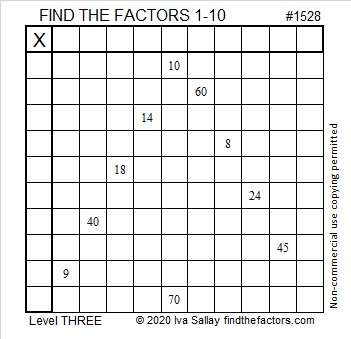# 1528 Candy Corn

Contents

### Today’s Puzzle:

To solve this Level 3 Candy Corn Halloween puzzle, first, find the factors that will work with the clues in the top and bottom rows. Then work you way down row by row filling in factors as you go.

When you get to the 8 in this puzzle, will the factors be 8 × 1 or 4 × 2? Two of those factors will be eliminated because they already appear in the first column. The other two remain possibilities, but one of those factors cannot appear in any other place in that first column, so that is the one you will want to choose. Have a sweet time solving this puzzle!Here is a plain version of the same puzzle:### Factors of 1528:

1528 is divisible by two because it is even.

1528 is divisible by four because its last two digits (in the same order) make a number, 28, which is divisible by 4.

Can 1528 be evenly divided by 8? Yes. Here’s a quick way to know: 28 is divisible by 4, but not by 8, AND 5 is odd, so 1528 is divisible by 8, as is every other number ending in 528.

• 1528 is a composite number.
• Prime factorization: 1528 = 2 × 2 × 2 × 191, which can be written 1528 = 2³ × 191.
• 1528 has at least one exponent greater than 1 in its prime factorization so √1528 can be simplified. Taking the factor pair from the factor pair table below with the largest square number factor, we get √1528 = (√4)(√382) = 2√382.
• The exponents in the prime factorization are 3 and 1. Adding one to each exponent and multiplying we get (3 + 1)(1 + 1) = 4 × 2 = 8. Therefore 1528 has exactly 8 factors.
• The factors of 1528 are outlined with their factor pair partners in the graphic below.### Another Fact about the Number 1528:

Since 1528 is divisible by 8 but not by 16, it can be written as the sum of 16 consecutive numbers:
88+89+90+91+92+93+94+95+96+97+98+99+100+101+102+103=1528.

Note that 95 + 96 = 191, and 8 × 191 = 1528.
Likewise, 94 + 97 = 1528,
93 + 98 = 1528, and so forth until we get to…
88 + 103 = 1528.

This site uses Akismet to reduce spam. Learn how your comment data is processed.